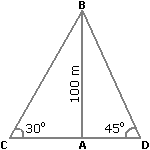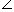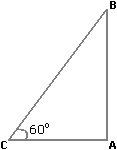# Topic: Basic Numeracy (Test 1)

Topic: Basic Numeracy
Q.1
A train 125 m long passes a man, running at 5 km/hr in the same direction in which the train is going, in 10 seconds. The speed of the train is:
A. 45 km/hr
B. 50 km/hr
C. 54 km/hr
D. 55 km/hr
Explaination / Solution:

 Speed of the train relative to man =125m/sec 10

 =25m/sec. 2

 =25 x 18km/hr 2 5

= 45 km/hr.

Let the speed of the train be x km/hr. Then, relative speed = (x - 5) km/hr.x - 5 = 45x = 50 km/hr.

Workspace
Report
Q.2
Two ships are sailing in the sea on the two sides of a lighthouse. The angle of elevation of the top of the lighthouse is observed from the ships are 30° and 45° respectively. If the lighthouse is 100 m high, the distance between the two ships is:
A. 173 m
B. 200 m
C. 273 m
D. 300 m
Explaination / Solution:

Let AB be the lighthouse and C and D be the positions of the ships.Then, AB = 100 m,ACB = 30° andADB = 45°.

 AB = tan 30° = 1AC = AB x √3 = 100√3 m. AC √3

 AB = tan 45° = 1AD = AB = 100 m. ADCD = (AC + AD) = (100√3 + 100) m = 100(√3 + 1) = (100 x 2.73) m = 273 m.

Workspace
Report
Q.3
The angle of elevation of a ladder leaning against a wall is 60º and the foot of the ladder is 4.6 m away from the wall. The length of the ladder is:
A. 2.3 m
B. 4.6 m
C. 7.8 m
D. 9.2 m
Explaination / Solution:

Let AB be the wall and BC be the ladder.Then,ACB = 60º and AC = 4.6 m.

 AC = cos 60º = 1 BC 2BC = 2 x AC = (2 x 4.6) m = 9.2 m.

Workspace
Report
Q.4
A sum of money at simple interest amounts to Rs. 815 in 3 years and to Rs. 854 in 4 years. The sum is:
A. Rs. 650
B. Rs. 690
C. Rs. 698
D. Rs. 700
Explaination / Solution:

S.I. for 1 year = Rs. (854 - 815) = Rs. 39.

S.I. for 3 years = Rs.(39 x 3) = Rs. 117.Principal = Rs. (815 - 117) = Rs. 698.

Workspace
Report
Q.5
Mr. Thomas invested an amount of Rs. 13,900 divided in two different schemes A and B at the simple interest rate of 14% p.a. and 11% p.a. respectively. If the total amount of simple interest earned in 2 years be Rs. 3508, what was the amount invested in Scheme B?
A. Rs. 6400
B. Rs. 6500
C. Rs. 7200
D. Rs. 7500
E. None of these
Explaination / Solution:

Let the sum invested in Scheme A be Rs. x and that in Scheme B be Rs. (13900 - x).

 Then,x x 14 x 2+(13900 - x) x 11 x 2= 3508 100 10028x - 22x = 350800 - (13900 x 22)6x = 45000x = 7500.

So, sum invested in Scheme B = Rs. (13900 - 7500) = Rs. 6400.

Workspace
Report
Q.6
The cost price of 20 articles is the same as the selling price of x articles. If the profit is 25%, then the value of x is:
A. 15
B. 16
C. 18
D. 25
Explaination / Solution:

Let C.P. of each article be Re. 1 C.P. of x articles = Rs. x.

S.P. of x articles = Rs. 20.

Profit = Rs. (20 - x).20 - x x 100 = 25x2000 - 100x = 25x

125x = 2000x = 16.

Workspace
Report
Q.7
In a certain store, the profit is 320% of the cost. If the cost increases by 25% but the selling price remains constant, approximately what percentage of the selling price is the profit?
A. 30%
B. 70%
C. 100%
D. 250%
Explaination / Solution:

Let C.P.= Rs. 100. Then, Profit = Rs. 320, S.P. = Rs. 420.

New C.P. = 125% of Rs. 100 = Rs. 125

New S.P. = Rs. 420.

Profit = Rs. (420 - 125) = Rs. 295.Required percentage =295 x 100% = 1475 % = 70% (approximately). 420 21

Workspace
Report
Q.8
Two students appeared at an examination. One of them secured 9 marks more than the other and his marks was 56% of the sum of their marks. The marks obtained by them are:
A. 39, 30
B. 41, 32
C. 42, 33
D. 43, 34
Explaination / Solution:

Let their marks be (x + 9) and x.

 Then, x + 9 = 56 (x + 9 + x) 10025(x + 9) = 14(2x + 9)3x = 99x = 33

So, their marks are 42 and 33.

Workspace
Report
Q.9
A fruit seller had some apples. He sells 40% apples and still has 420 apples. Originally, he had:
A. 588 apples
B. 600 apples
C. 672 apples
D. 700 apples
Explaination / Solution:

Suppose originally he had x apples.

Then, (100 - 40)% of x = 420.60 x x = 420 100x =420 x 100= 700. 60

Workspace
Report
Q.10
It was Sunday on Jan 1, 2006. What was the day of the week Jan 1, 2010?
A. Sunday
B. Saturday
C. Friday
D. WednesdayOn 31st December 2009, it was Thursday.